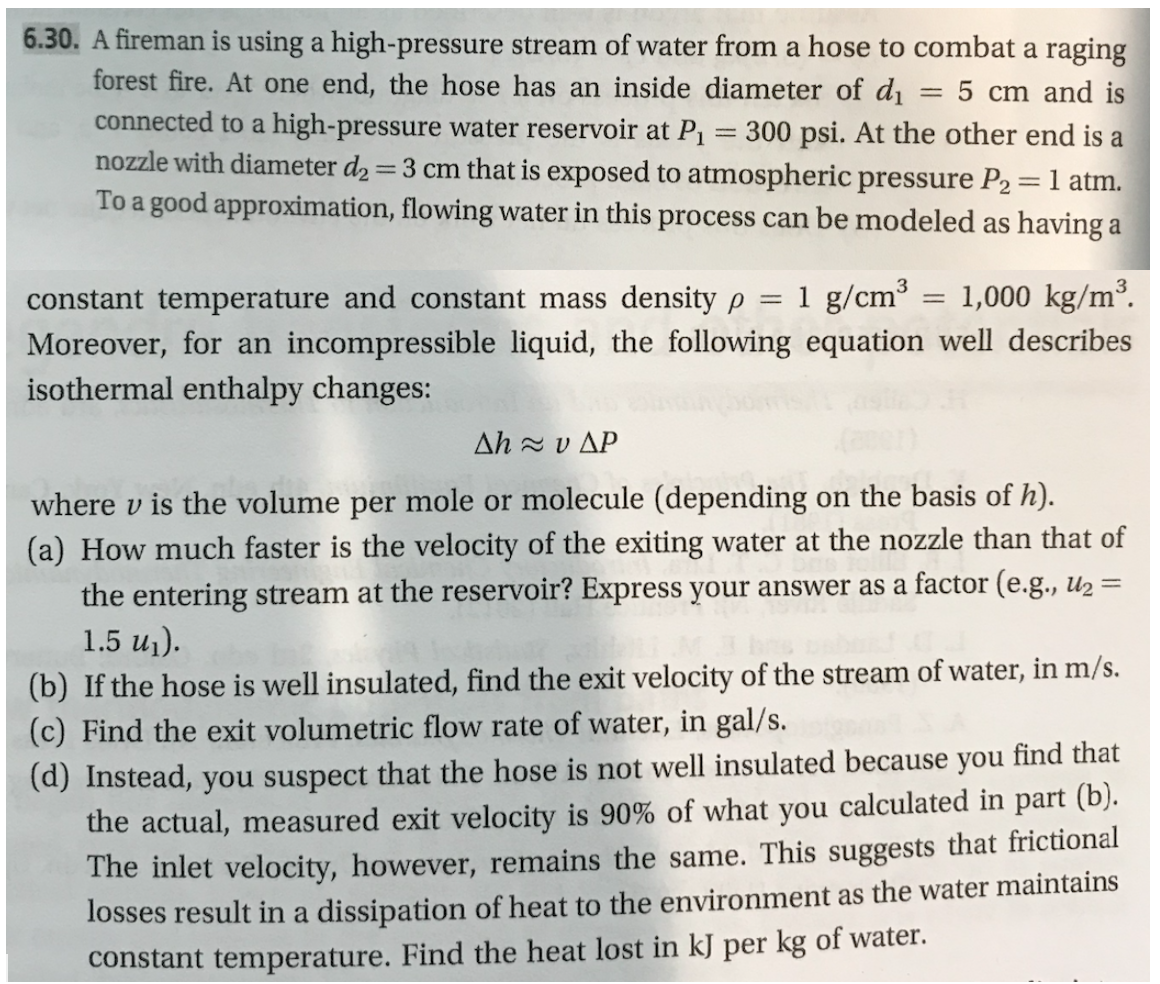# 6.30. A fireman is using a high-pressure stream of water from a hose to combat a ragingforest fire. At one end, the hose has an inside diameter of dconnected to a high-pressure water reservoir at P1 300 psi. At the other end is anozzle with diameter d2 3 cm that is exposed to atmospheric pressure P2= 1 atmTo a good approximation, flowing water in this process can be modeled as having a5 cm and is3constant temperature and constant mass density p = 1 g/cm = 1,000 kg/m2.incompressible liquid, the following equation well describesMoreover, for anisothermal enthalpy changes:Δh xυΔΡwhere v is the volume per mole or molecule (depending(a) How much faster is the velocity of the exitingon the basis of h)water at the nozzle than that offactor (e.g., u2=the entering stream at the reservoir? Express your answer as a1.5 u1).(b) If the hose is well insulated, find the exit velocity of the stream of water, in m/s.(c) Find the exit volumetric flow rate of water, in gal/s.(d) Instead, you suspect that the hose is not well insulated because you find thatthe actual, measured exit velocity is 90% of what you calculated in part (b).The inlet velocity, however, remains the same. This suggests that frictionallosses result in a dissipation of heat to the environment as the water maintainsconstant temperature. Find the heat lost in kJ per kg of water

Question
139 views

This problem is (6.30) from a book  "Thermodynamics and Statistical Mechanics An Integrated Approach by M. Scott Shell"help_outlineImage Transcriptionclose6.30. A fireman is using a high-pressure stream of water from a hose to combat a raging forest fire. At one end, the hose has an inside diameter of d connected to a high-pressure water reservoir at P1 300 psi. At the other end is a nozzle with diameter d2 3 cm that is exposed to atmospheric pressure P2= 1 atm To a good approximation, flowing water in this process can be modeled as having a 5 cm and is 3 constant temperature and constant mass density p = 1 g/cm = 1,000 kg/m2. incompressible liquid, the following equation well describes Moreover, for an isothermal enthalpy changes: Δh xυΔΡ where v is the volume per mole or molecule (depending (a) How much faster is the velocity of the exiting on the basis of h) water at the nozzle than that of factor (e.g., u2= the entering stream at the reservoir? Express your answer as a 1.5 u1). (b) If the hose is well insulated, find the exit velocity of the stream of water, in m/s. (c) Find the exit volumetric flow rate of water, in gal/s. (d) Instead, you suspect that the hose is not well insulated because you find that the actual, measured exit velocity is 90% of what you calculated in part (b). The inlet velocity, however, remains the same. This suggests that frictional losses result in a dissipation of heat to the environment as the water maintains constant temperature. Find the heat lost in kJ per kg of water fullscreen
check_circle

Step 1

The information provided in the question is as follows:

Inside diameter of the hose is d1 = 5 cm

Nozzle diameter is d2 = 3 cm

Pressure at the one end of the hose (let it be called as point 1) that is connected to a reservoir is P1 = 300 psi

The unit conversion for pressure is given as

1 atm = 14.7 psi

1 atm = 101325 Pa

So, P1 = 206785 Pa

At point 2, nozzle is exposed to the atmospheric pressure, i.e., P2 = 1 atm = 101325 Pa

Mass density ρ and temperature remains constant.

The isothermal enthalpy change is represented by relation Δh = vΔP, where v is the volume per unit mass, which can be taken as the reciprocal of mass density.

Step 2

Since the given system is an open system the energy balance applied between point 1 and 2 is given by equation (1) for a steady flow process.Here, Q is the heat transfer, W is the shaft work, ΔH is enthalpy change taking place, ΔK.E is change in kinetic energy and ΔP.E is change in potential energy.

Assuming the system is well insulated therefore, Q = 0. Also, no shaft work is obtained therefore, W= 0.  Since the hose and the nozzle are at the same level, therefore, z1 = z2 .Hence, ΔP.E = 0. So, equation (1) is reduced to equation (2).

Step 3

(a) According to the equation of continuity, the relation between the velocity of water in the hose (u1) and the nozzle (u2) is given by equation (3). In this equation A1 is the area of cross section of the hose and A2 is the area of cross section of the nozzle. A1...

### Want to see the full answer?

See Solution

#### Want to see this answer and more?

Solutions are written by subject experts who are available 24/7. Questions are typically answered within 1 hour.*

See Solution
*Response times may vary by subject and question.
Tagged in

### Fluid Mechanics